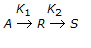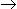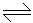# Chemical Engineering - Chemical Reaction Engineering

### Exercise :: Chemical Reaction Engineering - Section 9

36.

With increase in K2/K1 in case of a unimolecular type elementary reactions, the fractional yield of 'R' in mixed reactor (for a given conversion of 'A')

 A. decreases B. increases C. increases linearly D. remains same

Explanation:

No answer description available for this question. Let us discuss.

37.

Collision theory gives the rate constant for bimolecular reaction as

 A. K α T.e-E/RT B. K α eE/RT C. K α e-E/RT D. none of these

Explanation:

No answer description available for this question. Let us discuss.

38.

Find a mechanism that is consistent with the rate equation and reaction given below:
2A + BA2B, ( - rA) = k.CA.CB

 A. A + BAB ; AB + AA2B B. A + BAB;AB + AA2B C. A + AAA;AA + BA2B D. A + AAA;AA + BA2B

Explanation:

No answer description available for this question. Let us discuss.

39.

A gaseous reactant is introduced in a mixed reactor of 3 litres volume at the rate of 1 litre/second. The space time is __________ seconds.

 A. 1 B. 3 C. 1/3 D. 32

Explanation:

No answer description available for this question. Let us discuss.

40.

The temperature dependence of reaction rate constant (K) by Arhenius law is given by

 A. K α e-E/RT B. K α eE/RT C. K α T . e-E/RT D. K α T . e-E/RT